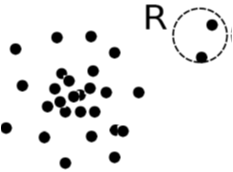### Categorization of Outlier Detection Methods

• Two ways to categorize outlier detection methods
• Based on whether user-labeled examples of outliers can be obtained
• Supervised methods
• Semi-supervised methods
• Unsupervised methods
• Based on assumptions about normal data and outliers
• Statistical methods
• Proximity-based methods
• Clustering-based methods

### Outlier Detection I: Supervised Methods

• Modeling outlier detection as a classification problem
• Samples examined by domain experts used for training & testing
• Methods for Learning a classifier for outlier detection effectively:
• Model normal objects & report those not matching the model as outliers, or
• Model outliers and treat those not matching the model as normal
• Challenges
• Imbalanced classes, i.e., outliers are rare:
• Boost the outlier class and make up some artificial outliers
• Catch as many outliers as possible
• recall is more important than accuracy (i.e., not mislabeling normal objects as outliers)

### Outlier Detection II: Unsupervised Methods

• Assume the normal objects are somewhat clustered'‘ into multiple groups, each having some distinct features
• An outlier is expected to be far away from any groups of normal objects
• Weakness: Cannot detect collective outlier effectively
• Normal objects may not share any strong patterns, but the collective outliers may share high similarity in a small area
• Ex. In some intrusion or virus detection, normal activities are diverse
• Unsupervised methods may have a high false positive rate but still miss many real outliers.
• Supervised methods can be more effective, e.g., identify attacking some key resources
• Many clustering methods can be adapted for unsupervised methods
• Find clusters, then outliers: not belonging to any cluster
• Problem 1: Hard to distinguish noise from outliers
• Problem 2: Costly since first clustering: but far less outliers than normal objects
• Newer methods: tackle outliers directly

### Outlier Detection III: Semi-Supervised Methods

• Situation: In many applications, the number of labeled data is often small: Labels could be on outliers only, normal objects only, or both
• Semi-supervised outlier detection: Regarded as applications of semi-supervised learning
• If some labeled normal objects are available
• Use the labeled examples and the proximate unlabeled objects to train a model for normal objects
• Those not fitting the model of normal objects are detected as outliers
• If only some labeled outliers are available, a small number of labeled outliers many not cover the possible outliers well
• To improve the quality of outlier detection, one can get help from models for normal objects learned from unsupervised methods

### Outlier Detection (1): Statistical Methods

• Statistical methods (also known as model-based methods) assume that the normal data follow some statistical model (a stochastic model)
• The data not following the model are outliers.
• Effectiveness of statistical methods: highly depends on whether the assumption of statistical model holds in the real data
• There are rich alternatives to use various statistical models
• E.g., parametric vs. non-parametric
• Example (below figure): First use Gaussian distribution to model the normal data
• For each object y in region R, estimate gD(y), the probability of y fits the Gaussian distribution
• If gD(y) is very low, y is unlikely generated by the Gaussian model, thus an outlier### Outlier Detection (2): Proximity-Based Methods

• An object is an outlier if the nearest neighbors of the object are far away, i.e., the proximity of the object is significantly deviates from the proximity of most of the other objects in the same data set
• 14
• The effectiveness of proximity-based methods highly relies on the proximity measure.
• In some applications, proximity or distance measures cannot be obtained easily.
• Often have a difficulty in finding a group of outliers which stay close to each other
• Two major types of proximity-based outlier detection
• Distance-based vs. density-based
• Example (right figure): Model the proximity of an object using its 3 nearest neighbors
• Objects in region R are substantially different from other objects in the data set.
• Thus the objects in R are outliers### Outlier Detection (3): Clustering-Based Methods

• Normal data belong to large and dense clusters, whereas outliers belong to small or sparse clusters, or do not belong to any clusters
• Since there are many clustering methods, there are many clustering-based outlier detection methods as well
• Clustering is expensive: straightforward adaption of a clustering method for outlier detection can be costly and does not scale up well for large data sets
• Example (below figure): two clusters
• All points not in R form a large cluster
• The two points in R form a tiny cluster, thus are outliersCreator: sidraaslam

Contributors:
-

Licensed under the Creative Commons
Attribution ShareAlike CC-BY-SA license

This deck was created using SlideWiki.